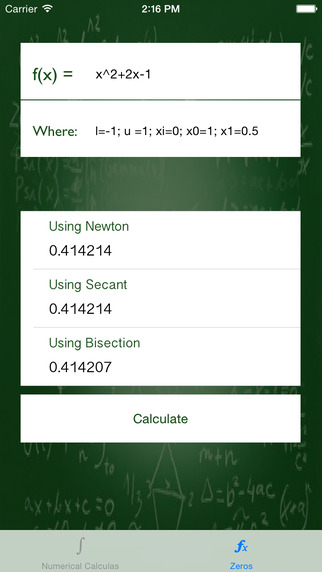# NumericalMathAs the name implies, this application uses Numerical Methods to Calculate some mathematical terms (So the answers are not exact, there is an error that the user can set).Definitions set:* For Derivative: Required: x (the value where the derivative would be calculated) example, x=2Optional: h (the step size), O(h^anyValue) (the upper bond of the error) example: x=2, h=0.001, O(h^6)* For Integrals:Required: l (lower bond), u(upper bond) example: l=0, u=2Optional: n(Number of iterations), O(h^anyNumber) example: l=0, u=2, n=5, O(h^4)* For Newton-Raphson methodRequired: xi (the initial guess) example: xi= 0Optional: e (the approximated error)example: xi=0, e=0.00001* For Secant methodRequired: x0 (the first guess) x1 (the second guess) example: x0= 0, x1=1Optional: e (the approximated error)example: x0= 0, x1=1, e=0.00001* For Bisection:Required: l (lower bond), u(upper bond) example: l=0, u=2Optional: e (the approximated error)example: l=0, u=2, e=0.0001This is the first release of NumericalMath application.This release supports the following:1- Calculating the value of the function at a given point2- Calculating the first derivative value of a function at a given point3- Calculating the integral value of a function in a given period4- Finds zeros of a function using Newton-Raphson method5- Finds zeros of a function using Secant method6- Finds zeros of a function using Bisection methodMissing:No user guide on how to use the system, in this version I'm assuming you know how to use it.Next release I'll provide proper guides.### 免費玩NumericalMath APP玩免費

#### 免費玩NumericalMath App

App Store
1.1
App下載

2015-05-162015-06-04